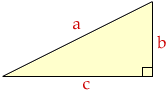Hi. I have a math exam tomorrow .What I ask you is this: can the sine /cosine rule be applied to a right -angle triangle to find a particular solution or are these 'rules' applied only when the triangle is not right angled? Hi Faydene, Both the law of sines and the law of cosines apply to any triangles, but for right angles triangles they don't add any useful information. In the diagram if the angle opposite a is a right angle then, since the cosine of 90o is zero, the law of cosines says that a2 = b2 + c2Also the sine of 90o is 1 so the law of sines for this triangle says  sin(a)/a =  sin(b)/b =  sin(c)/c or  1/a =  b/ab =  c/ac that is  1/a =  1/a =  1/a Cheers, Penny Go to Math Central